COMPLETED MAXWELL

ELECTROMAGNETIC FIELD THEORY (CEMT)

Nimit Theeraleekul B. Eng.

(June, 10, 2005, 4th revised - Feb, 1, 2012)

Abstract: Maxwell electromagnetic field theory originally was born with a mechanical model of the aether. After Einstein special theory of relativity was accepted, the aether was rule out. Only Maxwell equations are being used until now. Since then many important problems in electromagnetic field theory were started, and they still can not be solved until today. Modification using ōVacuum mechanicsö will solve all the problems and lead to a more completed theory of electromagnetic field!

Content: 1) Introduction. 2) Original problems in Maxwell electromagnetic field theory. 3) New model of Maxwell electromagnetic field theory. 4) Derivation of Maxwell equations. 5) Completed Maxwell electromagnetic field theory. 6) Electromagnetic waveÆs radiation process. 7) Electromagnetic waveÆs radiation resistance. 8) Mechanism of light wave. 9) Why light speed is constant? 10) What is photon rest mass? 11) Interaction mechanism between charges particles. 12) New interpretation of PoyntingÆs vector. 13) Conclusion. 14) References.

1) Introduction This article is the first expanding part of the original article ōVacuum mechanics a New Approach to the Theory of Everythingö (VMTE) . Because what has been talked there about Maxwell electromagnetic field theory (EMT) is rather a philosophical one, so in this paper it will be explained in a more scientific detail about the improvement of EMT. But before the improvement, it is necessary to summarize the main part of VMTE which will be involved with EMT as below.

1.1) Vacuum medium is the fabric structure of vacuum space. This is the fundamental hypothesis, or we could equally say that vacuum space was created from vacuum medium. So there is no such thing which was called as empty vacuum space (without anything), or there is no such thing like the old familiar aether that filled the empty vacuum space. Instead there is only vacuum space that coexists with vacuum medium, and we have called it as vacuum medium space! Indeed it is easy to prove its existence via a simple scientific experiment, i.e. a two-solenoid experiment .

In more detail, vacuum medium is a continuous isotopic homogenous medium which have a peculiar mechanical property. It is very thin in mass density that is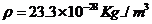(please see detail calculation in CGTR ), so it is permeable by all matters almost without any observed resistance! But it has very large elastic coefficient and sensitive to shear force (rotational force) while not to compressive or longitudinal force.

Technically, Vacuum medium is the geometrical structural gravitational potential energy; the energy that each of its infinitesimal part holds each other together by its internal attraction force (gravity) and forming to be the physical fabric structure of our space. If we interpret that the existing of vacuum medium space as positive energy and its internal attraction force as negative energy, then the total energy is zero. No vacuum medium without gravity means no space is existed; so the principle of conservation of energy is preserved!

1.2) Electrons are tiny black holes. This is the consequent hypothesis of vacuum mechanics. To be more precise, electrons are the condensed of vacuum medium in which each of its infinitesimal part holds each other together by its intrinsic gravitation force! Indeed we could prove this hypothesis using theoretical verification by solving the problem of electronÆs mass . By using the same argument as electron, we would found that proton is also a tiny black hole but (with different size) a heavier one.

Now, what followed from the above hypothesis is that when an electron or proton is staying still, under this situation, it will attach the surrounding vacuum medium (because it is something like black hole). And this will create the internal stress in the vacuum medium around, which is appeared as electrostatic field. But when electron is moving, it will rotate in the direction parallel to the motionÆs axis. So it will create rotational stress in the surrounding vacuum medium while dragging it along, this is magnetostatic field!

1.3) Vacuum mechanics -- a new conceptual mechanism for all natural phenomena. In physics, when we deal with rigid solids, we use the mechanics of rigid bodies as the tool. And when dealing with liquids or gases what we used is fluid mechanics. In the same way, we will use vacuum mechanics - the mechanism of vacuum medium when dealing with vacuum medium. This include the interaction between condensed vacuum medium (electron and proton) with vacuum medium, also the interaction between condensed vacuum medium themselves.

2) Original problems in Maxwell electromagnetic field theory. When James Clerk Maxwell first created the electromagnetic field theory, he also invented a mechanical model of elastic solid (aether) called a granular model of space  for its philosophical concept. May be because the aether concept was so strange and the model was too complicated. And the most important point is that (at that time) no one could prove the existing of the aether! So after Einstein had created special theory of relativity (STR) which no need the aether, then people have ignored the proposed model and only the equations were used until now.

Now, we could see that the theory is limited to abstractions which using only the mathematical formula to describe it. It is not a physical theory because there is no philosophic idea behind for explaining how the theory works! So how could we understand the theory without its mechanism? While we know that ōunderstandingö is the progress of science, without it we could be mislead to something crazy. Indeed we will see later that this is the starting point of the problems which will follow then.

Let us start with some familiar phenomena of electromagnetic waves. First, look for the mechanism of electromagnetic wave propagation. It is well known that all type of waves require some physical mediums to be the carrier which behave as the mechanism for their propagation! But after STR had rule out the existence of the aether that was used as the mechanism of waves, the problem was started to occur.

Most of conventional text books have tried to explain that electromagnetic wave could propagate by the mechanism of itself, that is, by mutual creation between changing of electric field and changing of magnetic field. But if we look more carefully, we will found that it contradicts to the principle of causality because both of the generated electric and magnetic field are created simultaneously (and also changing at the same instance) by their common source; time-variable electric current .

Next problem is about electromagnetic waves radiation process. Most of standard text books have the difficulty for clearly explanation how the process works . Other more problem in electromagnetic field theory is about ōradiation resistanceö that will occur during the radiation process. If we want to send radio signal via an antenna, we have to feed electric power into the radio antenna to overcome the radiation résistance. The question is what resistance that we have to overcome? This was asked by Nobel Prize laureate Richard P. Feynman . Actually when we study more detail and deep in electromagnetic field theory, we would found more and more the questions could not be answered. And some of them will be discussed later.

Up to now, from the above discussion we could get some clue of the problems, i.e. it is because we have ōnothingö to deal with; ōsomethingö which acts as the mechanism of every phenomenon that occurs in electromagnetic field theory.  Someone may ask, are we going back to old aether theory? The answer is yes and no.  Yes, we are returning to the general concept analogous to the concept of the old aether. But no, it is not the old ether that filled vacuum space which we want to talk about, but it is the vacuum space medium itself!

Here, armed with the new concept of vacuum mechanics - the mechanism of vacuum medium, we will show how to solve all the problems mentioned above. Under the concept of vacuum space medium summarized in section 1, two essential parts are involved in electromagnetic field theory, i.e. condensed vacuum medium ¢ electron (which play the role of tiny black hole), and vacuum medium.

3) New model for Maxwell electromagnetic field theory. Now, we have two main ingredients which are involved in electromagnetic field i.e. vacuum medium and electron. While electron (charge particle) is the generator of electromagnetic field, vacuum medium is the mechanism for transporting the field energy. So now we are ready to create an appropriate mechanical model for the theory. The new model proposed is the process for flowing of direct current in a simple electric circuit as show in the diagram fig. 1(a) below.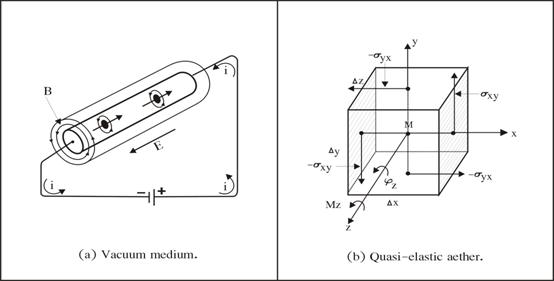Figure 1. New model for Maxwell electromagnetic field theory.

The new model will follow the same concept of ōthe quasi-elastic body model of aetherö fig. 1(b), which was developed in 19PPPPPPPPPPPthPPPPPPPPPPP century by Arnold Summerfeld . The reason because the aether has the same mediumÆs mechanical property as our vacuum medium, i.e. they are both continuous elastic medium which are sensitive to rotation.  What we will concentrate here in the new model proposed in fig. 1(a) is the flowing stream of electrons (-i) pass through the conducting wire with negligible resistance (for simplicity). This was done by electromotive force provided from battery and which also maintaining potential difference for the wire.

Remember that the moving stream of electrons will generate electric field along the wire. And because they also rotate while dragging the surrounding vacuum medium around the wire, this will create the rotational stress in vacuum medium which is appeared as surrounding magnetic field B (or H according to the reference). In a consequent at the same time the torsion wave was generated and propagates along the wire at a speed of light.

By the way, someone may worry about the slow flowing speed of electronsÆ current, and how its effect could occur with speed of light. One way of explanation is using analogy with pressure wave occurred at the outlet of waterÆs pipe when we first turn on the valve . Anyway, it is not so good one; because it seems to occur only at the instant which the valve was turn on.

A better analogy for explaining the paradox is to use a row of several pieces of metal balls hanging from a horizontal bar. If we start to swing the first ball in the row, and when it impact the next (second) ball, at that instant the third, the fourth and the remaining ones will stay still for awhile. A second later, the last ball will respond the transfer series of impact by swing away from the row.

In the analogy, metal balls represent electron particles. When the first electron was driven by an external electromotive force and moving slowly, it will impact the second electron, then the second electron will impact the third electron and so on. With the series of the impact, the last electron will respond as if it is the first electron moving with faster speed. In conclusion the slow moving electrons could give a faster action as it was appeared!

4) Derivation of Maxwell equations. Now we will apply the derivation of the quasi-elastic body model of aether show in fig. 1 (b) to our vacuum medium space. First letÆs start with the volume element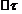of the body be twisted through the angle φ with a moment,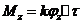ģģ (a)                (Where k = twist modulus of vacuum medium)

Because the quasi-elastic body is sensitive to rotation and the rotation has the character of an antisystemmatic tensor. Then we get shear stresses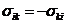ģģģģ (b)               (Where i, k run for x, y and z)

From (a) and (b), stress-rotation relation in the figure is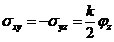ģģģ. (c)

The moments originate at the two z-surface is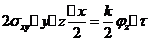ģģģ.(d)

And the moments originate at the two y-surface is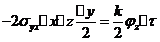ģģģ.(dÆ)

So the total moment is in fact that of (a). By rotation of the letters we obtain from (c)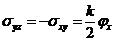and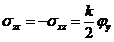ģģģ.(e)

Now we got the equation of the quasi-elastic body of vacuum medium as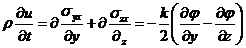.

Or in vector form,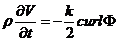ģģ  (f)      (Where ρ = vacuum medium density)

This is the equation of motion of vacuum medium. When it is supplemented by the relation between the velocity v and the angular velocity w which reads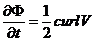ģģģģģ (g).  (Where w =d Φ / d t was replaced by Φ / t.)

With the additional assumption for the incompressibility of the vacuum medium and note that Φ, being the curl of the displacement vector, has no divergence. Thus v and Φ are subject to the condition

div v = 0     ģģģ(h)           div Φ = 0 ģģģģ (k)

From Maxwell equations in free space, we get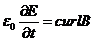ģ. (fÆ),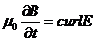ģģ(gÆ)

div E = 0 ģģģ.ģ (hÆ)                 div B = 0     ģģģ(kÆ)

Comparing (f), (g) (h) and (k) to (fÆ), (gÆ) (hÆ) and (kÆ), we found that they are identical! And if we continue to work out more, we will finally get the result - the velocity of light in the vacuum medium as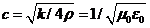ģģģ. (m)

(Where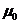= permeability and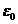= permittivity of vacuum medium.)

Next to get the complete equations for our new model, we must include electric current density j from energy source which provide the applied toque. And referenced to the explanation for electrostatic field and magnetostatic field in section 1.2, then for electrostatic field (internal stress in the surrounding vacuum medium) that was created from electric charge q (tiny black hole). According to Gauss law, then we could interpret it as

div E = q    ģģģģ..(n)            (where q = enclosed charge)

From AmpereÆs law, the magnetostatic field (rotational stress in the surrounding vacuum medium) is created from the moving electric charge (tiny black hole). This could interpret it as

curl B = j ģģģģ..   (o)   (where j = electric current density)

When we combine (fÆ) and (o) then what we got is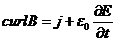ģģ.(p)

Finally, we could see that equations (gÆ), (kÆ), (n) and (p) are the complete set of Maxwell equations!

5) Completed Maxwell electromagnetic field theory. Up to now we have improved the conventional Maxwell electromagnetic field theory to be a more complete theory which is more rational and understandable. Also we could see that all electromagnetic field phenomena are the appearances of the dynamics action between electron and vacuum medium. It is a part of the mechanism of vacuum medium (vacuum mechanics), and so we should call it as ōvacuodynamicsö! Now we are ready to solve the problems in the electromagnetic field theory mentioned early.

5.1) Continuous wave vs. wave packet. Before going into detail with electromagnetic waves, let us talk about one of its important characteristic.  Normally when dealing with electromagnetic waves which include radio waves, light wave etc., it seem at first that all are continuous wave as shown in diagram fig. 2(a) below. But if we observe carefully we would found that there is also a short portion of wave train which was called ōwave packetö as shown in fig. 2(b).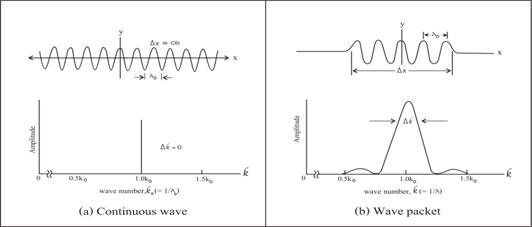Figure 2. Two types of natural waves.

Note that a continuous wave has a sharply defined wavelength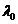and a corresponding sharply defined wave number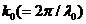. There is nothing about this wave that suggests the localization in space that associate with the word ōparticleö.  Instead, wave packet could be created by adding sine wave with properly chosen wave numbers, amplitudes and phases.

This collection of infinite waves adds to make a sine wave over a certain region of width ∆x and, by destructive interference, adds to zero everywhere else. The prize we have paid is the sacrifice of the ōpurityö of our original wave because the packet now no longer contains a single wave number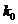but rather a spread of wave numbers center about.

For mechanical wave such as water wave, it is easy to see both types of waves. Wave packet could be created by applying a short duration of the disturbance source such as dropping a stone in water. But if we dip a vibrating folk in water, the created waves will be a continuous wave train.

For electromagnetic waves, there are both continuous waves and wave packets. Normally radio waves which are man-made waves are continuous waves, while light waves which are occurred in nature are wave packets which were called as ōphotonsö.

Next sections we will see how continuous electromagnetic wave was generated by manmade, while light wave packet was created by nature, i.e. the mechanism of light!

6) Electromagnetic waveÆs radiation process. For the new model in section 4, we have seen how the flowing stream of electrons creates magnetic field around and electric field the conducting wire. Now if the supply power source is a high frequency generator instead of a battery as show in the diagram fig. 3(a). The flowing up and down of electronÆs stream will generate waves in vacuum medium around the antenna conductor and then moving away from the wire as shown in fig. 3(b). This is the radiation process of electromagnetic waves.

In more detail, the antenna conductor used above is a half-wave (of the radio frequency) length and which was called a half wave dipole radio antenna. So the overall electromagnetic waves power radiation field pattern is a donut shape as shown in fig. 3(c). In the next section we show how to calculate the total power of radiation field pattern.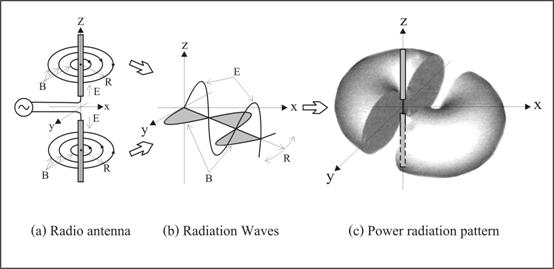Figure 3. Electromagnetic waves radiation process.

7) Electromagnetic waveÆs radiation resistance. Up to now we could see that electromagnetic wave was created by disturbance of vacuum medium around the antenna, and then propagating away. It is the oscillation; the dynamic stress ¢ stain relation within vacuum medium. To do this, some power must be used to overcome the radiation resistance due to the resistive action of vacuum medium. And to find the value of the radiation resistance of the antenna, we have to calculate its radiation power first as below.

Mathematically we say that the radiated electromagnetic field is the tensor field (stress tensor) or PoyntingÆs vector of vacuum medium. Below is the summary for its derivation referenced to the book . (Actually we could found it in most text book for radio communication.)

We start with the supplied current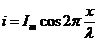to the antenna as shown in fig. 4(a). [Where x has values from 0 to ± λ/4.] The magnetic field at P due to current i in the element dl is,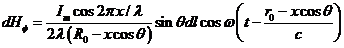ģ.. (1)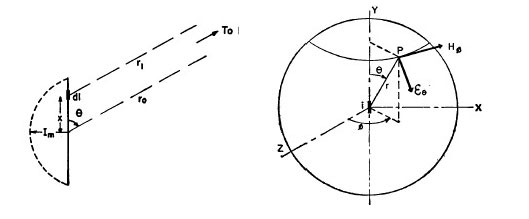(a) Half-wave dipole antenna.                   (b) Coverage of radiation field.

Figure 4. Radiation field of half-wave dipole antenna.

Then the total magnetic field at P is,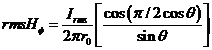ģģģ (2)

Also the total electric field at P is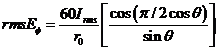ģģģģ (3).

Finally the total electromagnetic field at P is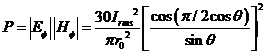ģ (4).

This is the calculated total value of radio waves radiation pattern for a half wave dipole antenna as shown in fig. 4 (b). Then the total power W radiated from the antenna could be found by integrating the close surface for P, and the result is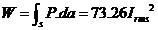wattsģģģģ. (5).

Finally, to get the radiation resistance, because the calculated power in any electrical circuit is equal to the resistance times the square of flowing current, then for a half-wave dipole antenna in (5), the radiation resistance is 73.26 ohms. (Actually the true value is a little higher due to the resistance of the antenna conductor.)

8) Mechanism of light wave. Now we will talk about light wave packet ¢ photon, in which we have learnt that conventionally it is emitted from an exited atom. Anyway, it still not clears how it works in detail. And to see the problem, let us start with the conversation between Richard P. Feynman and his father in ōThe Physics Teacherö (September 1969) about light  as follow;

[My father] said, ōI understand that they say that light is emitted from an atom when it goes from one state to another, from exited state to a state of lower energy.ö I said, ōThatÆs right.ö

ōAnd light is a kind of particle, a photon, I think they called it.ö I said, ōYes.ö

ōSo if the photon comes out of the atom when it goes from the exited to the lower state, the photon must have been there in the exited state?ö I thought a few minutes and I said, ōI am sorry; I donÆt know. I canÆt explain it to you

What we learnt from the conversation is the knowledge that ōfrom where that light is originatedö and also the problem that ōhow light is originatedö! Now we will discuss in more detail as follow.

First of all, let us start with the topic ōmomentum of lightö by Richard P. Feynman [6Æ] as follow. In diagram fig. 5(b), suppose light is coming from a source and is acting on an electron charge q with its electric field E component and driving it up and down with velocity v. Then magnetic field B component (which is right angle to the electric field E) will act on the moving electron and create the resultant force F. This driving force F is in the direction of the propagation of light and was called ōradiation pressureö or light pressure!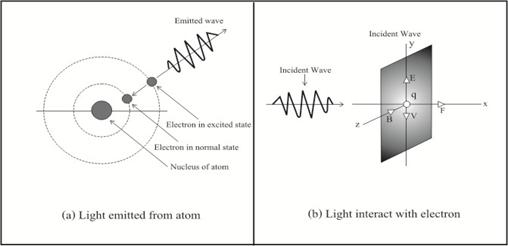Figure 5. Mechanism of light waves.

Next we know that the radiation pressure is F = qvB. But because everything is oscillating, then the radiation pressure is also the time average of F is < F>.  And because B = E/c, so <F> = q<vE>/c! Remember that the charge q times the field E is the electric force on the charge, and the force on the charge time the velocity v is the work dW/dt being done on the charge! Therefore the ōpushing momentumö that is delivered per second by the light, is equal to 1/c time the energy absorbed from the light per second!

In any circumstance where light is being absorbed, there is a pressure. The momentum that light delivers is always equal to the energy that is absorbed, divided by c. And we already know that light carries energy. Also we now understand that it is also carries momentum, and further, that the momentum carried is always 1/c time the energy as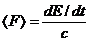[Here we replaced the symbol of the energy W with E]

Finally when light is emitted from a source there is a recoil effect: the same thing in reverse. If an atom is emitting an energy E in some direction, then there is a recoil momentum p = E/c.

Now, we are ready to explain how light is emitted from an atom by using vacuum medium wave packet. In diagram fig. 5(b), when an electron (in an atom) was hit by a photon (or any other kinds of electromagnetic waves which energy is big enough), it will moves from normal state of lower energy to excited state of higher energy. After the collision, the exited electron will jump back from unstable excited state to its normal lower stable state immediately. Because the ōtransition timeö is very short (1.6 nanosecond for hydrogen atom ), then the jumping back electron will transfer its impulse forceÆs energy to the surrounding vacuum medium. This makes the disturbed vacuum medium oscillates and radiate out as a ōwave packetö of light wave which was called photon as shown in fig. 5(a)! (Note that this is the reverse process mentioned in the previous paragraph.)

9) Why light speed is constant? In section 4 the velocity of light in the vacuum medium was derived as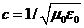ģģģ. (m),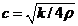ģģģ. (mÆ)

(Where= permeability and= permittivity of vacuum, k and ρ are twist modulus and density of vacuum medium.)

We knew from Maxwell electromagnetic field theory that bothandin (m) are empirical constant. But both of them are just the proportional constant that occurred in Maxwell equation (fÆ) and equation (gÆ), in which it does not tell us why they must be constant!

Instead, according to the concept of vacuum medium which was assumed that it is isotopic and homogenous, so both k and ρ are constant. Then what follow from (mÆ), is that the velocity of light wave c must also be constant! This is the same thing as the velocity of other natural wave, such as sound wave for example, which is constant due to the uniform density of the medium air.

Finally, we often heard that vacuum medium should be very rigid for creating such a huge speed c of light! This idea comes from the concept that sound speed in rigid medium such as steel which is greater than the non-rigid medium such as air, for example. But this is not quite right; the better method is to compare the same kind of medium, such as normal air and hydrogen. For airÆs mass density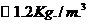which has sound speed 331m/s, while hydrogen mass density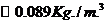has sound speed 1284m/s. The reason is because mass density of hydrogen is lighter than the air (assumed both have the same modulus), so its sound speed is higher than air according to wave speed formula (m) which equal to square root of modulus over density. And because vacuum mediumÆs density is very dilute, so it is not surprise that light wave speed in vacuum medium must be very high speed!

10) What is photon rest mass? Again this is another problem of photon which was arisen because modern physicists do not accept that light (photon) is the wave packet of vacuum medium.  Armed with our new concept, it is easy to understand the reason why photon has no rest mass while it cannot stop from traveling (propagation) with speed c.

As mentioned early that all kind of natural wave is the vibration of some kind of physical medium, for example sound wave is the vibration of air. And it is easy to understand that a pulse of sound wave (analogous to photon) also has no rest mass too. The reason is because the vibration of air create sound wave that traveling from its source; staying still of sound wave means no vibration or no sound wave (then no rest mass exist). Indeed, all other kind of natural wave such as water wave, including light wave (photon) cannot stop (no vibration) and has no rest mass!

11) Interaction mechanism between charge particles. Up to now, in order to give us some confidence in the concept that charge particles are tiny black holes (mentioned early), we will use it to explain how the interaction between charge particles work. Conventionally ōdifferent type of charge particles attract, while the same type of charge particles repelö, but there is no explanation why?

Armed with the concept of tiny black hole, it is easy to understand the interaction, but in a different view which is more reasonable, that is ōdifferent size of charge particles attract, while the same size of charge particles repelö. Now let us see how the interaction mechanism works.

11.1) Repelling between same size charges. Actually all sizes of black holes must attract each other. This is what Richard P. Feynman told in his book , in which he observed that proton and proton attract each other when they are separate at some distance apart! But when they are close together less than a certain distance then they will repel each other, anyway he did not say why it is so!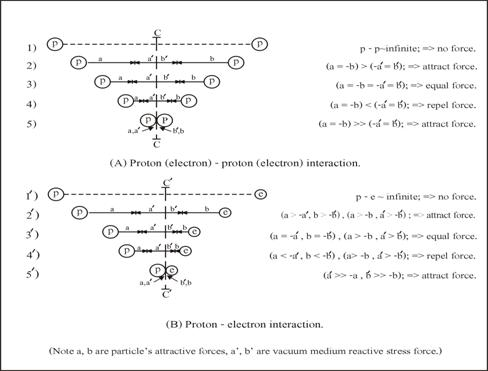Figure 6. Interaction mechanisms between charges particles.

The reason that there are two types of the interaction between charge particles (tiny black holes) is because they all are immerged in vacuum medium, and how the existing of vacuum medium is the cause of the repulsion between the same sizes of tiny black hole which will be explained below.

According to diagram fig. 6(A) it is the interaction between proton and proton or between electron and electron. Cases (1) when the separate distance is infinity, no interaction force between them appear. Case (2) when they are closing together, they begin to attract each other because both of them are tiny black holes. Case (3) at a certain distance where the attractive forces of the black holes is equal to the repulsive force of the internal stress in vacuum medium between them (because black holes create internal stress in the vacuum medium around), then they stop to attract each other. Cases (4) when the separated distance is closer than in (3), then they repel each other. This is the phenomenon we are familiar, i.e. same type of charges repel! Case (5) when both particles are forced to stick together, that is the attract force of the black holes is greater than the reaction force of the internal stress in vacuum medium. This is how two or more proton particles could stay in an atom nucleus.

(Note, the vertical line c ¢ c is perpendicular and divide into two half of the line joint between p and p, while the line cÆ ¢ cÆ in (B) is closed to e than p due to their different size.)

11.2) Attraction between different size charges. In (B), it is the interaction between proton and electron. Case (1Æ) and (2Æ) are the same situation as (1) and (2) in (A). Case (3Æ) is the same as in (3) (A), but the only different is the separate distance between p and e which is smaller than in (3) (A). The reason is because of the different size between proton and electron. And this is the phenomenon we are familiar, i.e. different type of charges attract. Case (4Æ), when the separated distance is closer than in (3Æ), then they begin to repel each other as in case (3) (A). Case (5Æ), it is the same situation as (5) in (A), and what we got is a neutron!

12) Problems involved PoyntingÆs vector. Poynting vector S, is a very important concept when we work with the flowing of electromagnetic field energy. It tells us the rate at which the field energy moves around in space. Indeed all radio wave transmission energy is calculated using this concept as was shown in section 7!  Anyway, when working with it in some particular cases, we would found that we are facing with some crazy problems [11Æ]!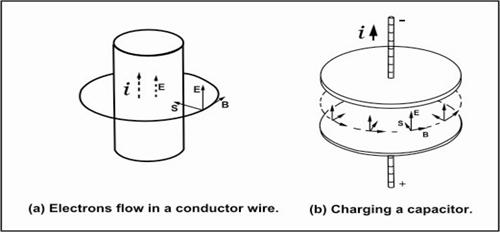Figure 7. Problems involved PoyntingÆs vector.

According to the conventional concept of Poynting vector, such as heat dissipation energy in a conductor wire, it is not come from the flow-in electron current; instead it comes from the field energy around the wire as show in diagram fig. 7(a). Another example is the charging energy to store in a capacitor is not come from the flow-in electron current; instead it comes from the field energy around the capacitor as show in diagram fig. 7(b). These problems arise because of the ambiguity of the field, i.e. we do not know what really electromagnetic field is!

12.1) Conventional interpretation of PoyntingÆs vector. Before solving the problems by using our new concept of vacuum mechanics, we will first see detail how modern physics understand (or ¢ not understand?) and work out the electromagnetic field energy by using Poynting vector concept as follow.

First let us consider diagram fig. 7(a), which shows a current i flow through a conductor wire length l, with internal resistance R. And to produce the current, we need an electric field E parallel to the wire of magnitude iR/l.  Also we have learnt early that when electrons (current) move, they will also rotate and create magnetic B around the wire according to the application of Biot ¢ Savart law i.e.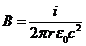[Where r is the wire radius of the wire]

Now according to Poynting vector,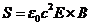is the energy (per unit area per second) flows in the wire from outside around the wire.

Then the total energy around the wire which flows in it is equal to the heat dissipate from the wire resistance,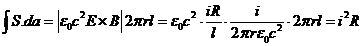So it was concluded that the heat dissipate from the wire resistance is not coming from the input current, instead it comes from the flow in energy around the wire!

Next let us consider diagram fig. 7(b), a capacitor being charged slowly. There is a nearly uniform electric field inside which is changing with time. At any instant the total electromagnetic energy in the capacitor is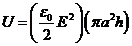ģ (1).

(Where a = radius, h = plateÆs separation of the capacitor.)

And the rate of receiving energy of the capacitor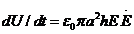ģ (2)

Now when the capacitor is charging, there is a magnetic field that circulates around the axis. And by using one of Maxwell equations, the circulate magnetic field is given by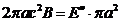, then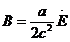ģģ (3).

Using Poynting vector (S), it seems that the receiving energy is flowing in from space around. Then the rate of the flowing in energy through the surface area of 2¶ah is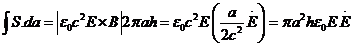ģ (4).

That is, it is equal to (2). And conventionally it was explained that the flowing of electromagnetic field energy into the capacitor comes from space around it (not the current from input). Of course we knew that it is not true!

12.2) New interpretation of PoyntingÆs vector. To solve the problems involved Poynting vector concept, first let us first go back to our proposed new model of Maxwell electromagnetic field which is the process for flowing of direct current in a simple electric circuit as show in the diagram fig. 1(a) in section 3.

Remember that electrons are tiny black holes, so when they are moving they will rotate and drag the surrounding vacuum medium around the wire. This will create rotational stress in vacuum medium which is appeared as the surrounding magnetic field B.  Also since the wire has resistance, then there is an electric field along it for driving the current. And because there is a potential drop along the wire, there is also an electric field E just outside the wire and parallel to the surface.

Now come to the crucial point which mislead to the crazy problems mentioned above; it is the direction of Poynting vector S which point toward the wire, and then it was interpreted as the energy flow into the wire. The reason of the misinterpretation is because modern physicists do not understand what really the electromagnetic field energy is!

To solve the problem of the conductor wire, it is easy by using our new concept that electrons are tiny black holes. Because the electrons will attract the surrounding vacuum medium and creating compressive rotational stress in the medium, which is then manifest as electromagnetic energy around the wire. And according to the compressive rotational stress, its direction is point toward into the wire, not outward as the conventional interpretation. In conclusion, the flow-in current (of electrons) give rise to the dissipate heat energy while create the surrounding electromagnetic energy around the wire!

Finally, to solve the problem of the capacitor, we will use our new concept that electrons are tiny black holes in the same way as was done with the conductor wire. But because the dissipate heat energy and the surrounding electromagnetic energy around the connecting wire is small (compare to the capacitor energy) so we will neglect them.

When the flow-in current of electrons accumulates in the upper disc plate of the capacitor, they will create something like a rotating disc of tiny black holes. Then the rotating disc will drag and rotate the vacuum medium between the two plates and forming a compressive rotational stress in the medium, in which it appears as the flow-in electromagnetic field energy as show in the diagram. In conclusion, the flow-in current give rise to the energy store in the capacitor, while creates a compressive rotational stress in the vacuum medium between the two plates in which it appeared as the flow-in electromagnetic field energy into the capacitor!

13) Conclusion. Up to now we have shown how to improve Maxwell electromagnetic field theory, i.e. we have added a suitable model to fulfill its philosophical concept. Then the theory is not just a mathematical equations, but it is what we could understand how it work. So the existing problems which born in the conventional theoretical concept could be eliminated by using the new concept of vacuum mechanics!

Actually we could see that all electromagnetic field phenomena, i.e. electrostatic field, magnetic field and electromagnetic field are the appearances of the mechanism of vacuum medium ¢ vacuum mechanics. More specific, it is the interaction between electron (condensed vacuum medium) and vacuum medium. So more properly, we could say that the action of electrodynamics is the action of ōvacuodynamicsö - the dynamic of vacuum medium!

People who are familiar with Einstein special theory of relativity would know that STR was born in order to solve some problems occurred in electrodynamics that was not work properly in the realm of Newton classical mechanics. Anyway, we will found (in the article ōCompleted Einstein special theory of relativity (CSTR) ) that STR cannot solve all the problems in EMT, instead it give rise to some fundamental problem which we talked early!

Anyway we could also found that what was done in this paper is not fully mathematical rigor. But it provides some detail just enough for us to have some confidence in the new proposed concept. And it is the starting point for modification of the theory. So from now on, it is time for the professional which is involved to complete it!

14) References.

(Precaution; Several text books with different authors were used as the reference and each author used different pattern and different notation for the same formula. Here in this paper, the author has made some change of the original pattern and notation for the readers convenient, so please be careful in reading!)

 By Nimit Theeraleekul, now presenting in www.vacuum-mechanics.com

 Nimit Theeraleekul, ōöCompleted Einstein general theory of relativity (CGTR)ö, now

presenting in www.vacuum-mechanics.com

 James Blake Westgard, ōElectrodynamics: A Concise Introductionö p. 90-95.

 Oleg D. Jefimenko, ōCausality Electromagnetic Induction and Gravitationö Second Edition

p. 13-16.

 Constantine A. Balanis ōAntenna Theory, Analysis and Designö p.7-11.

 Richard P. Feynman, Robert B. Leighton and M. Sands, ōThe Feynman Lectures on

Physicsö. Vol. 1, p.32-1 to 32-2, for [6Æ] p. 34-10 to 34-11.

 Arnold Sommerfeld, ōMechanics of Deformable Bodiesö, p.108 ¢ 111.

 D. Halliday, R. Resnick, and K. Krane, ōPhysicsö Vol. 2 Extended Version Fourth Edition,

p.700.

 John D. Ryder, ōNetwork, Lines and Fieldsö Second Edition, p.535-548.

 Peter W. Milonni,öThe Quantum Vacuum, (An Introduction to Quantum

Electrodynamics)ö, p. 77.

 John S. Townsend ōA Modern Approach to Quantum Mechanicsö p.435.

 Richard P. Feynman, Robert B. Leighton and M. Sands, ōThe Feynman Lectures on

Physicsö Vol. 2, p. 8-7, for [11Æ] p. 27-6 to 27-8.

 By Nimit Theeraleekul, now presenting in www.vacuum-mechanics.com

ģģģģģģģģģģ.